# Python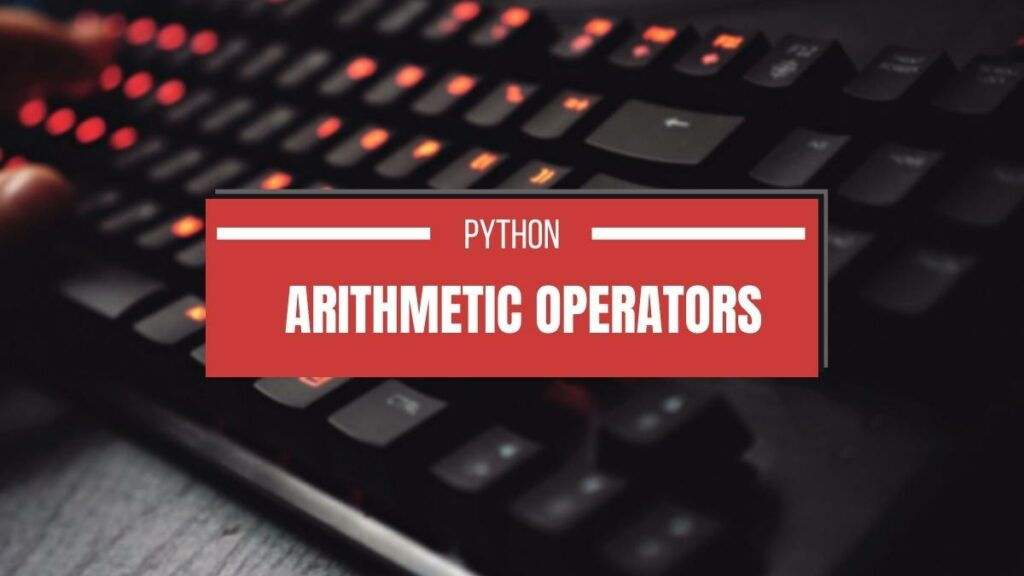## Arithmetic Operators in Python [With Examples]

In this tutorial, you will learn about arithmetic operators in Python with examples. In Python, you can use arithmetic operators to perform Mathematical operations such as addition, multiplication, division, etc. Python arithmetic operators are binary, which means that it requires two operands to operate.  The value on which the operators operate is known as operands. …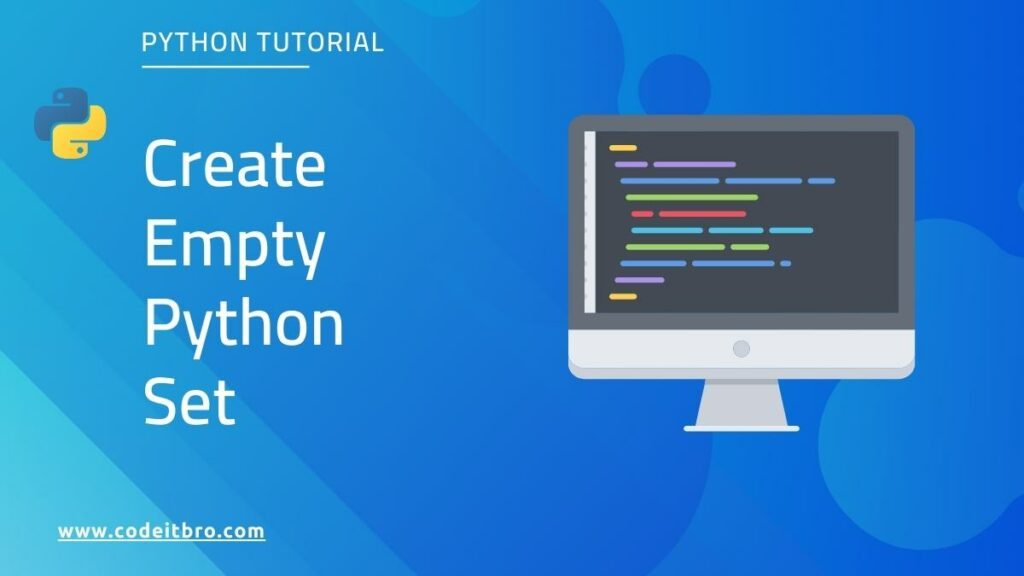## How to Create Python Empty Set

This tutorial will discuss how to create a Python empty set and different operations performed on the set. In Python, a set is a data structure that will store unique elements. It will not allow duplicates. Set is represented by {}. Example: my_set={1,2,3,4,5} Also Read: Python Matrix Using Numpy [With Examples] How to Create Python Empty …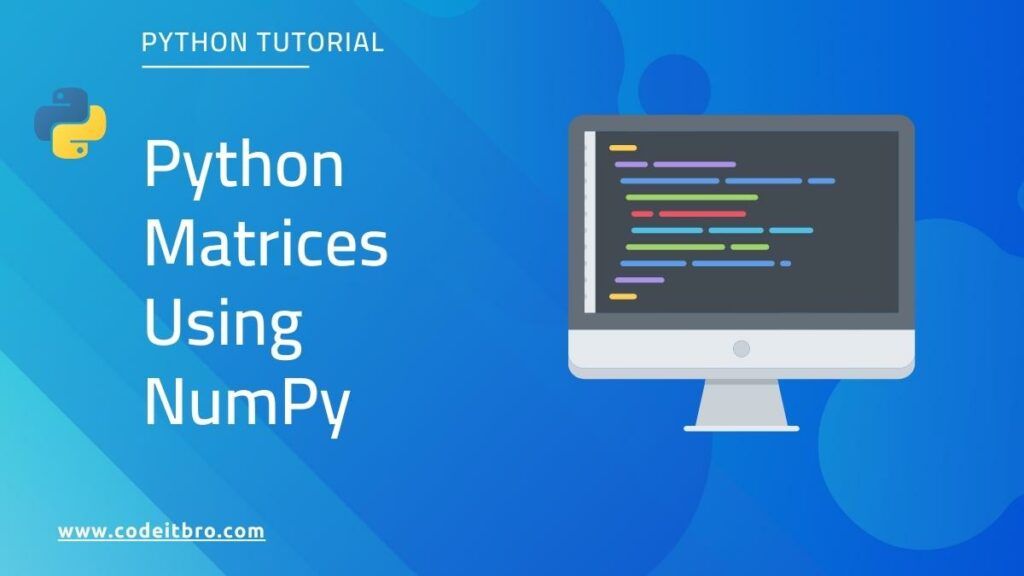## Python Matrix Using Numpy [With Examples]

In this Python tutorial, we will learn all about matrices using the NumPy package. We will see how to create a matrix using NumPy arrays and various methods/operations performed on the matrix, such as adding or removing a row/column. A matrix is a data structure where data is stored in rows and columns, as shown …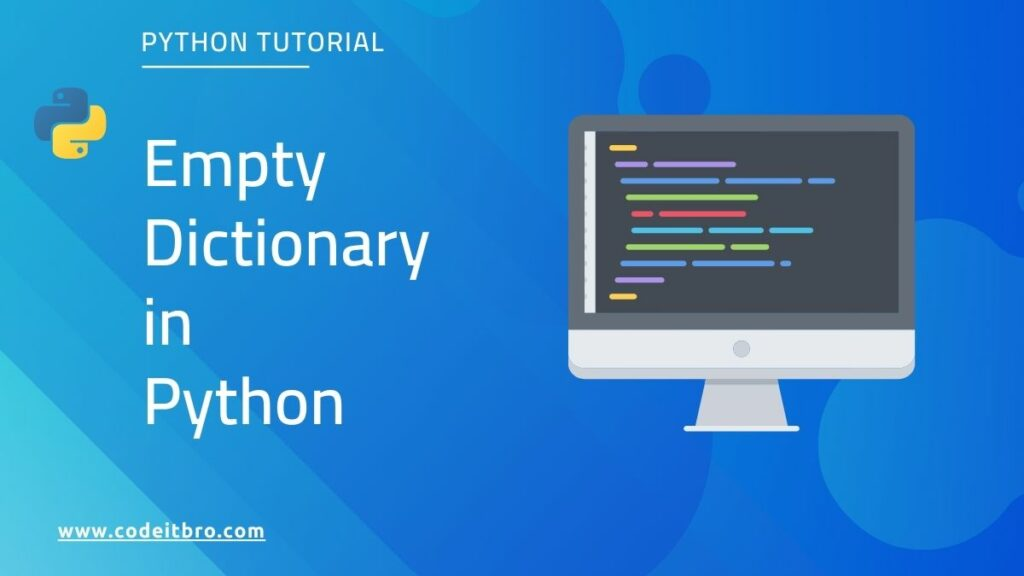## How To Create An Empty Dictionary in Python

Dictionary in Python stores data as key-value pairs. In this article, we will discuss how to create an empty dictionary in Python and various Dictionary methods such as copy(), get(), update(), etc. A dictionary in Python is a changeable collection, ordered, and does not allow duplicates. Keys will be unique, and their values can be …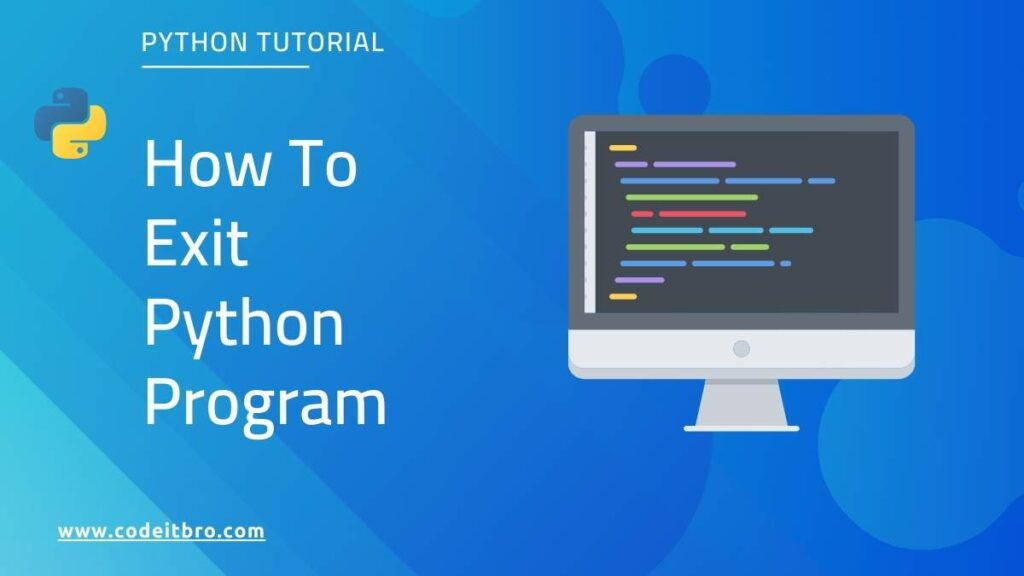## How to Exit Python Program [4 Methods]

A Python program gets terminated as soon as the interpreter reaches the end of the file. However, we can also terminate a Python script using various exit commands. This tutorial will discuss how to exit Python program and different ways to terminate it. To exit a Python program, we will explore the following methods: Quit() …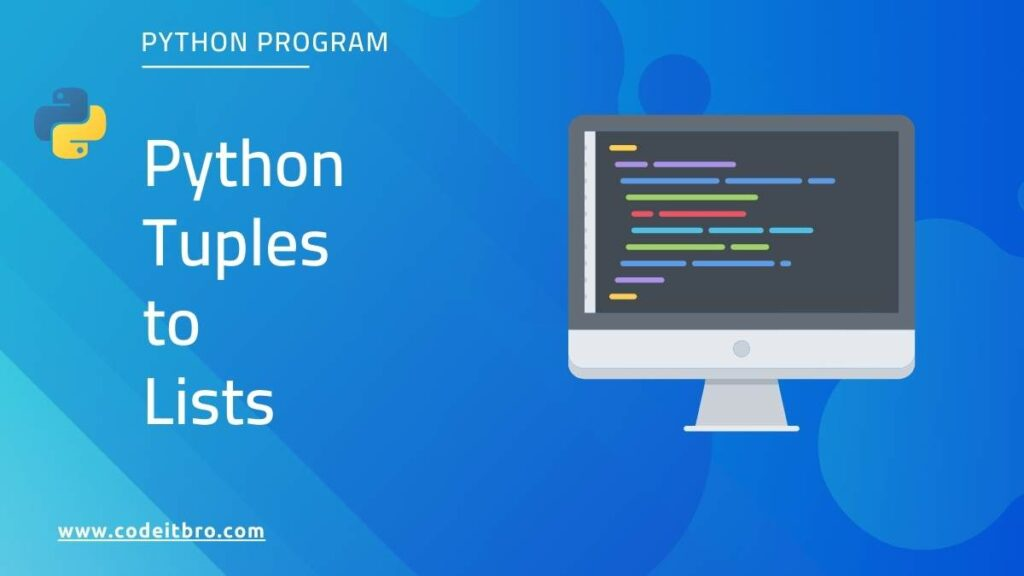## How to Convert Python Tuples to Lists

This tutorial will discuss how to convert Python tuples to lists. We have covered four methods using which you can use to convert tuples to lists in Python: List() function unpack (*) operator Using list comprehension Map() function Also Read: How to Handle String Index Out of Range Error In Python What is a Tuple in …## How to Handle String Index Out of Range Error In Python

In this tutorial, we will discuss how to handle string index out of range error. Before moving ahead, let’s first understand what is string index out of range error is in Python. Just like lists, strings are also indexed in Python. We can define a string as a set of Unicode characters stored inside. In …## How to Reverse an Array In Python [Flip Array]

This article will discuss how to reverse an array in Python. Interestingly, there is no in-built array data structure in Python. Instead, it comes with lists that offer more robust data storage features and operations. However, we can use the conventional arrays in Python using either array or NumPy libraries. So, our tutorial is divided …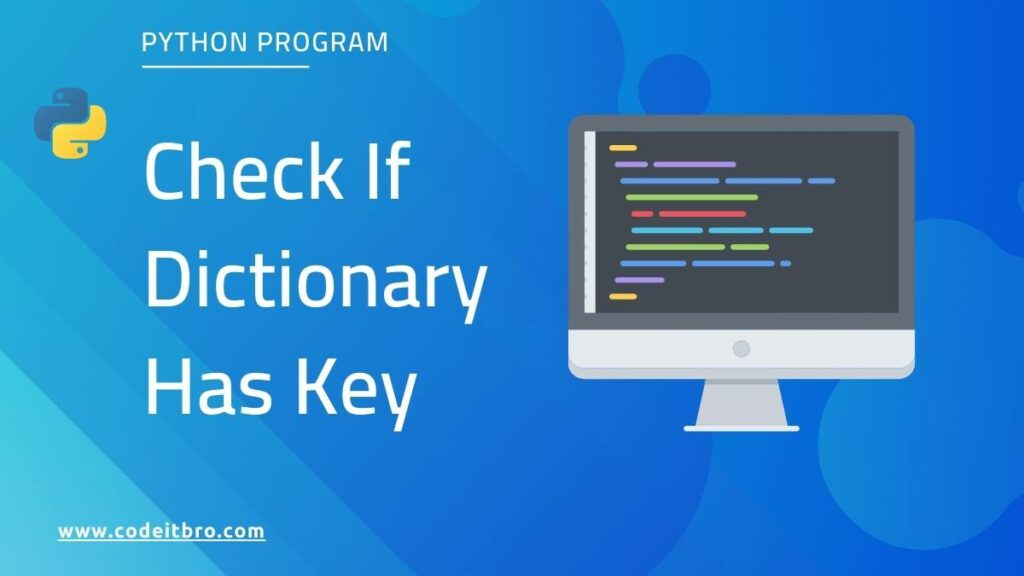## How to Check If Dict Has Key in Python [5 Methods]

In this tutorial, you will learn how to check if the dictionary has a key in Python. We have covered five methods to search single or multiple keys in a dictionary. A dictionary in Python stores data in key-value pairs. During programming, you might encounter scenarios where you would have to fetch data from a …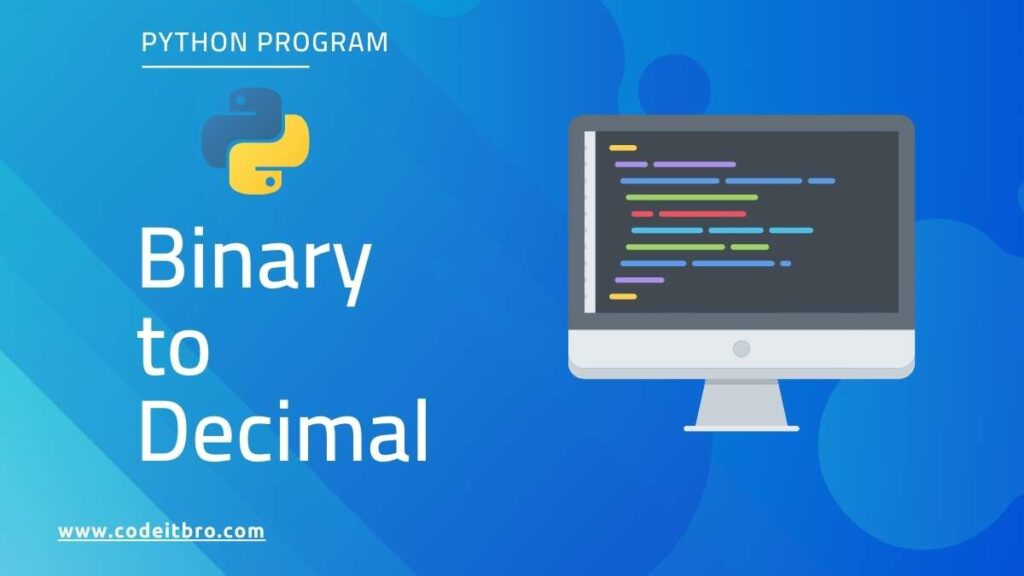## How to Convert Binary to Decimal in Python [5 Methods]

This article will discuss how to convert binary to decimal in Python. So there are different methods to convert binary to decimal in Python. A binary number is represented in the form of 0’s and 1’s, and a decimal number is in the form of an integer value. How to Convert Binary to Decimal in …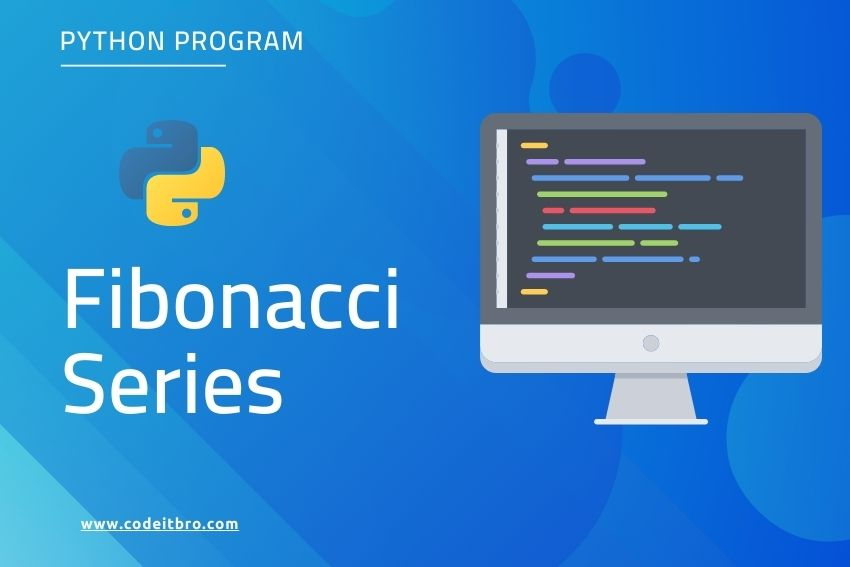## Python Program To Display Fibonacci Series Up To N Terms

In this Python program, you will learn how to display Fibonacci sequence or series up to N terms. Before moving ahead, you should be aware of the following basic concepts of the Python programming language: Variables and operators For loop While loop If…else statement Must check resources for Python: 10 Best Books To Learn Python …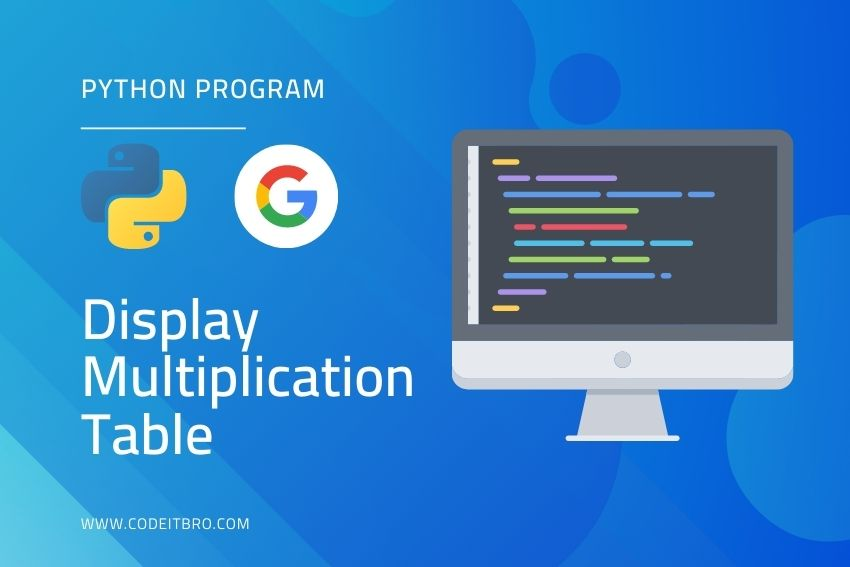## Python Program To Display Multiplication Table of Any Number

In this Python code tutorial, you will learn how to display the multiplication table of any number. Before moving ahead, please ensure that you have a basic knowledge of the following concepts: Python syntax Variables and operators For loop Before moving ahead, you must check these resources for Python beginners: 10 Best Books To Learn …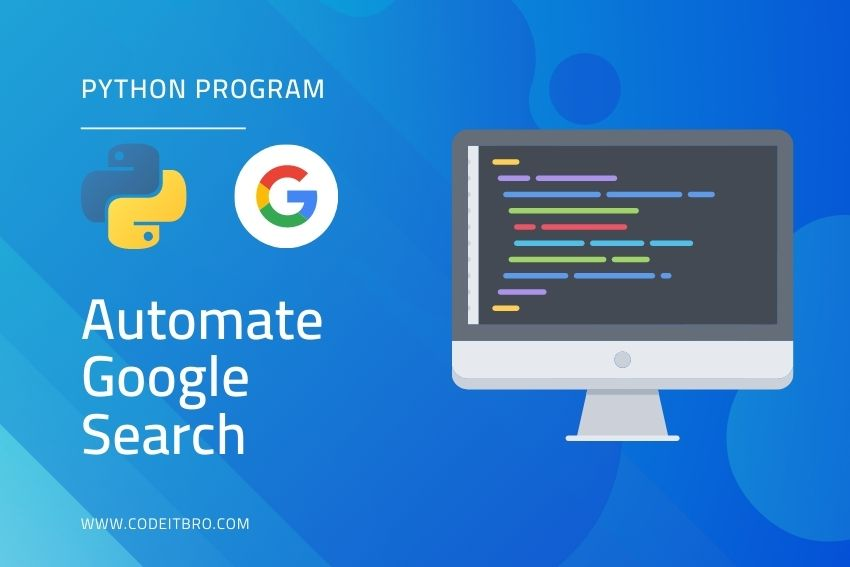## How To Automate Google Search With Python

This tutorial will learn how to automate Google Search with Python. To perform Google using Python code, we will use multiple libraries, making it easier for you to scrape search results. The first library we will use to perform Google searches is googlesearch-python and the second one is google. These Python libraries will make scraping …

Scroll to Top